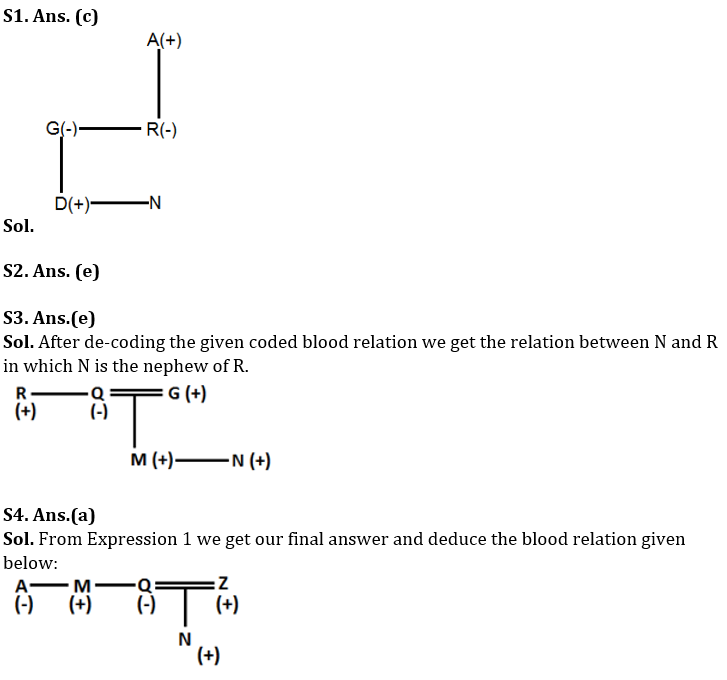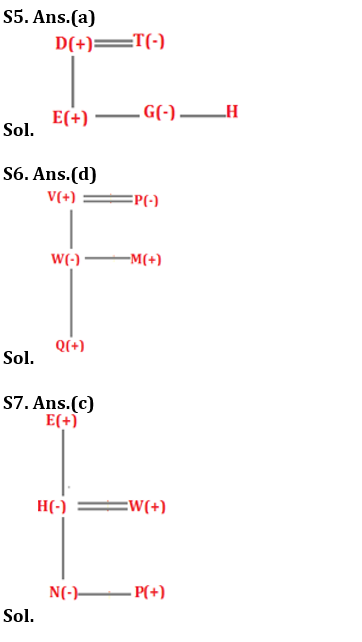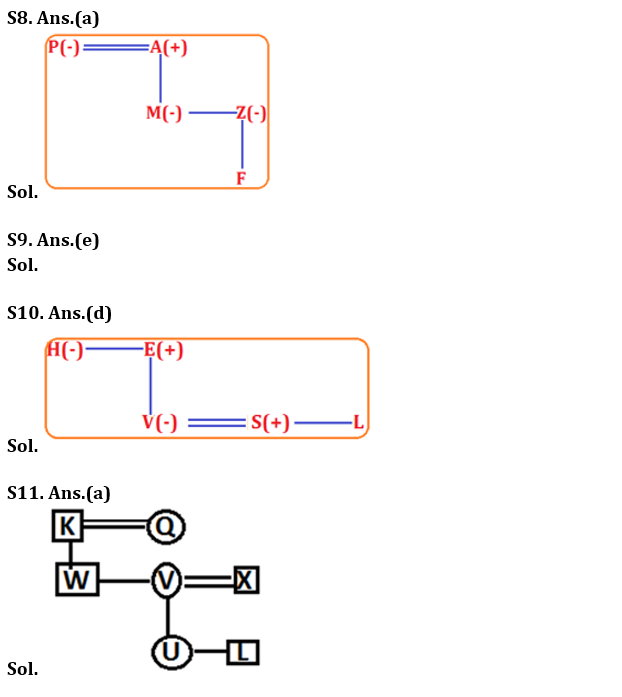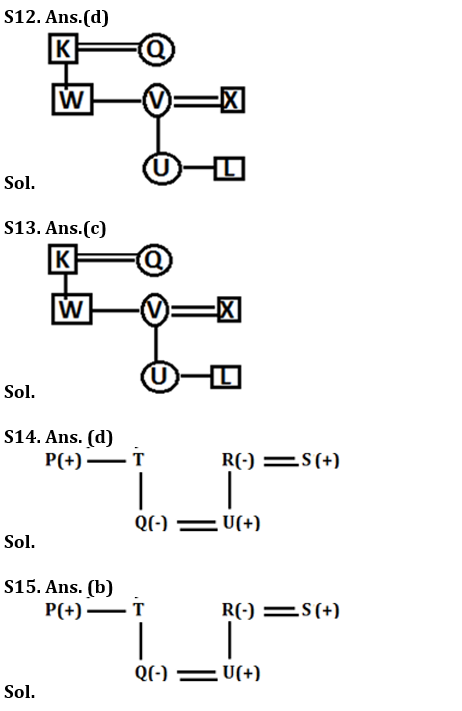Latest Banking jobs   »   Reasoning Ability Quiz For IBPS Clerk...

Reasoning Ability Quiz For IBPS Clerk Prelims 2021- 14th December

Directions (1-2): Following questions are based on the information given below.
‘P × Q’ means ‘P is father of Q’
‘P – Q’ means ‘P is sister of Q’
‘P + Q’ means ‘P is mother of Q’
‘P ÷ Q’ means ‘P is the brother of Q’

Q1. In the expression A × R –G + D ÷ N, how is D related to A?
(a) Granddaughter
(b) Son
(c) Grandson
(d) Brother
(e) None of these

Q2. Which of the following represents ‘Q’ is son of ‘U’?
(a) Q ÷ I – G × U
(b) Q + I – G × U
(c) Q ÷ M – N × U
(d) Q ÷ J – N + U
(e) None of these

Directions (3-4): Following questions are based on the information given below.
‘P × Q’ means ‘P is Son of Q’
‘P – Q’ means ‘P is sister of Q’
‘P + Q’ means ‘P is mother of Q’
‘P ÷ Q’ means ‘P is brother of Q’

Q3. In the expression R ÷ Q + M ÷ N × G, how is N related to R?
(a) Granddaughter
(b) Son
(c) Niece
(d) Granddaughter or Grandson
(e) None of these

Q4. In which of the following expression Q is wife of Z?
(a) A–M÷Q+N×Z
(b) A–Q÷M+N×Z
(c) Q–M÷A+N×Z
(d) Q–R÷A÷N×Z
(e) None of these

Directions (5-7): These questions are based on the following information:
‘A @ B’ means ‘A is father of B’.
‘A \$ B’ means ‘A is mother of B’.
‘A * B’ means ‘A is sister of B’.
‘A # B’ means ‘A is daughter of B’.
‘A = B’ means ‘A is son of B’

Q5. How is D related to H, in the given expression ‘D @ E = T \$ G * H’?
(a) Father
(b) Mother
(c) Sister
(d) Son
(e) None of these

Q6. Which of the following statements is/are true, if the given expression Q = W # V @ M = P is true?
(a) Q is father of M
(b) V is husband of P
(c) P is grandmother of Q
(d) Both (b) and (c) are true
(e) All are true

Q7. What is the relation between E and W in the given expression?
E @ H \$ N * P = W
(a) E is father of W
(b) E is daughter of W
(c) E is father-in-law of W
(d) E is brother of W
(e) None of these

Directions (8-10): Study the following information carefully and answer the questions which follow.
‘M + K’ means ‘M is brother of K’
‘M ÷ K’ means ‘M is father of K’
‘M × K’ means ‘M is wife of K’
‘M – K’ means ‘M is sister of K’
‘M = K’ means ‘M is mother of K’

Q8. In expression ‘P × A ÷ M – Z = F’, how is F related to P?
(a) Grandchild
(b) Grandson
(c) Granddaughter
(d) Grandmother
(e) None of these

Q9. Which of the following expression represents the relationship ‘D is daughter – in – law of C’?
(a) D × H + N = E ÷ C
(b) H + D × N ÷ E = C
(c) C = F + L – Z × D
(d) C × F ÷ L ÷ Z × D
(e) None of these

Q10. In equation ‘H – E ÷ V × S + L’, how is E related to S?
(a) Mother-in-law
(b) Mother
(c) Niece
(d) Father-in-law
(e) Cannot be determined

Directions (11-13): Study the following information to answer the given questions.
There are seven members of a family of three generations and two are married couples. K is the father-in-law of X. W is the only son of Q. There are two children of V, who is married to brother-in-law of W. U is not the Son of X. L is the grandson of the husband of Q.

Q11. Who is the maternal grandmother of U?
(a) Wife of K
(b) V
(c) W
(d) L
(e) Mother of L

Q12. Who is the daughter of Husband of Q?
(a) W
(b) X
(c) L
(d) V
(e) U

Q13. How is W related to U?
(a) Grand father
(b) Father
(c) Maternal Uncle
(d) Niece
(e) Nephew

Directions (14-15): Study the following information carefully to answer the given questions below.
P is the brother of T. Q is the daughter of T. R is the mother-in-law of Q. R is married to S. U is the only son of S. R has only one child.

Q14. How T is related to U?
(a) Father-in-law
(b) Mother-in-law
(c) Uncle
(d) Can’t be determined
(e) Aunt

Q15. How Q is related to P?
(a) Nephew
(b) Niece
(c) Uncle
(d) Can’t be determined
(e) Aunt

Solutions×

Thank You, Your details have been submitted we will get back to you.Join India's largest learning destination

What You Will get ?

•Job Alerts
•Daily Quizzes
•Subject-Wise Quizzes
•Current Affairs
•Previous year question papers
•Doubt Solving session

ORJoin India's largest learning destination

What You Will get ?

•Job Alerts
•Daily Quizzes
•Subject-Wise Quizzes
•Current Affairs
•Previous year question papers
•Doubt Solving session

ORJoin India's largest learning destination

What You Will get ?

•Job Alerts
•Daily Quizzes
•Subject-Wise Quizzes
•Current Affairs
•Previous year question papers
•Doubt Solving session

Enter the email address associated with your account, and we'll email you an OTP to verify it's you.Join India's largest learning destination

What You Will get ?

•Job Alerts
•Daily Quizzes
•Subject-Wise Quizzes
•Current Affairs
•Previous year question papers
•Doubt Solving session

Enter OTP

Please enter the OTP sent to
/6

Did not recive OTP?

Resend in 60sJoin India's largest learning destination

What You Will get ?

•Job Alerts
•Daily Quizzes
•Subject-Wise Quizzes
•Current Affairs
•Previous year question papers
•Doubt Solving sessionJoin India's largest learning destination

What You Will get ?

•Job Alerts
•Daily Quizzes
•Subject-Wise Quizzes
•Current Affairs
•Previous year question papers
•Doubt Solving session

Almost there

+91Join India's largest learning destination

What You Will get ?

•Job Alerts
•Daily Quizzes
•Subject-Wise Quizzes
•Current Affairs
•Previous year question papers
•Doubt Solving session

Enter OTP

Please enter the OTP sent to Edit Number

Did not recive OTP?

Resend 60

By skipping this step you will not recieve any free content avalaible on adda247, also you will miss onto notification and job alerts

Are you sure you want to skip this step?

By skipping this step you will not recieve any free content avalaible on adda247, also you will miss onto notification and job alerts

Are you sure you want to skip this step?